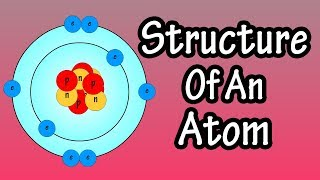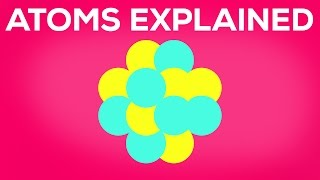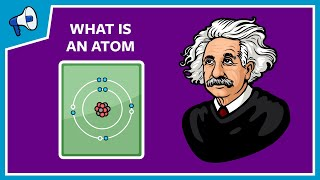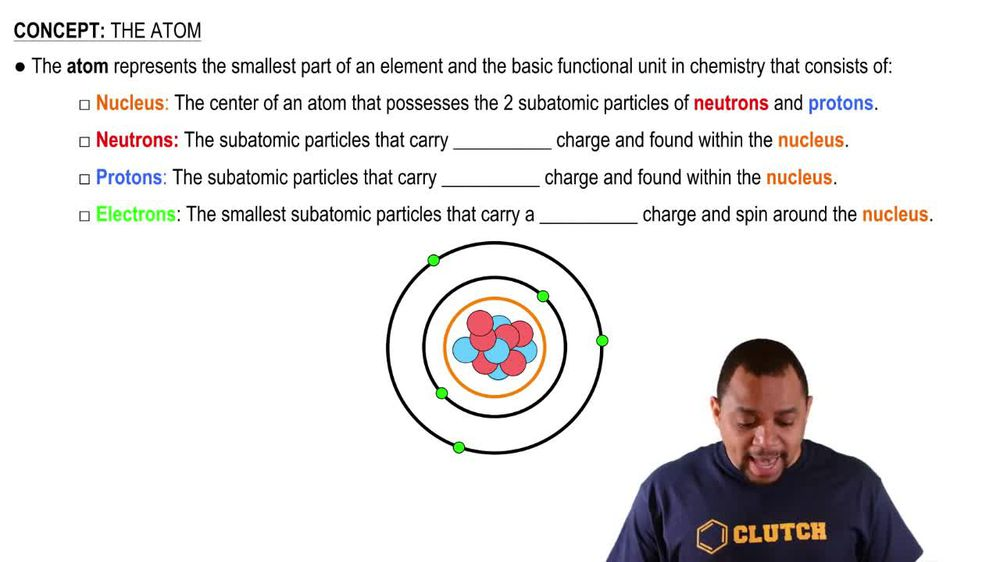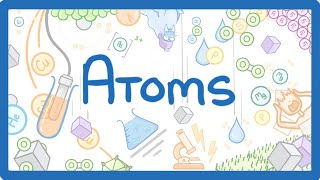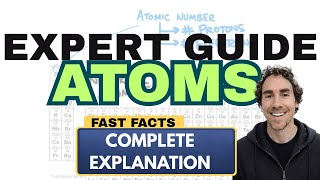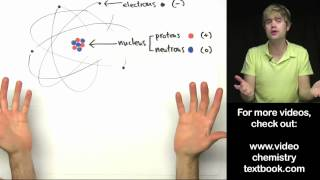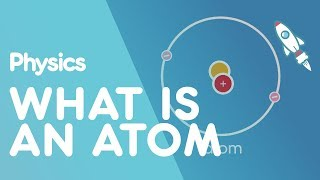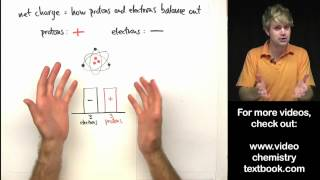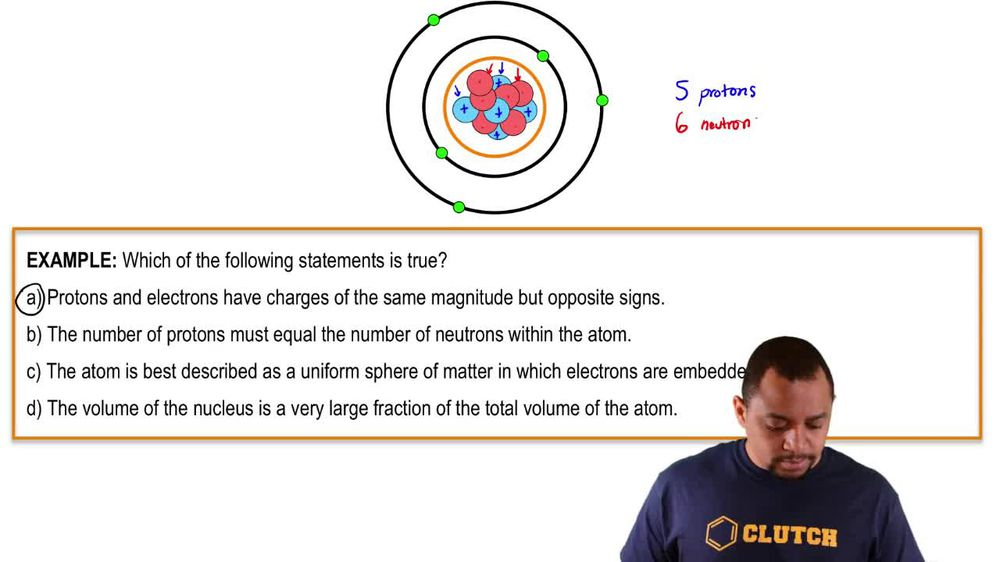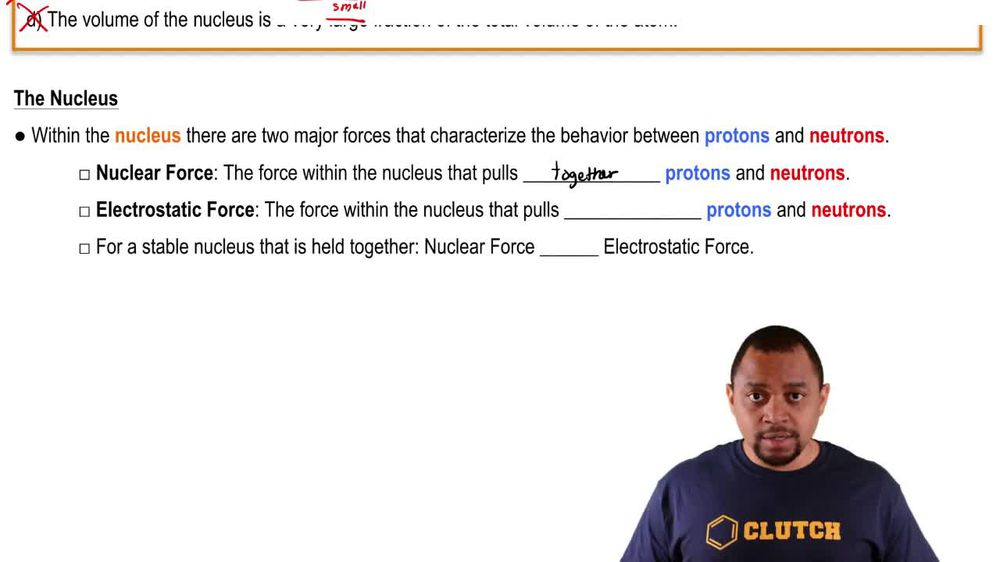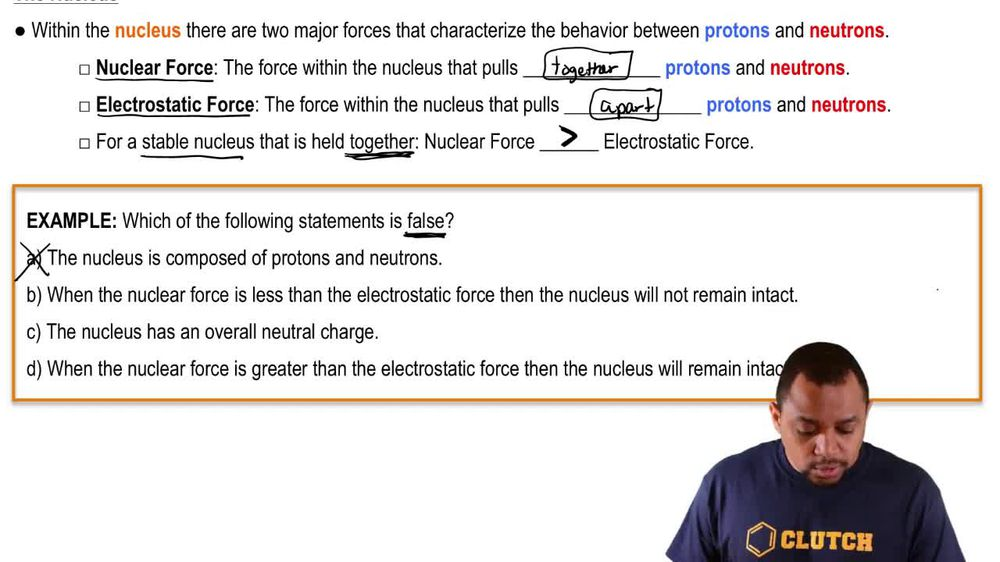Start typing, then use the up and down arrows to select an option from the list.
1. 2. Atoms & Elements2. The Atom
Problem

# Determine whether each of the following statements is true or false. If false, correct the statement to make it true: (d) The protons in the nucleus of the helium atom are held together by a force called the strong nuclear force.

Relevant Solution2m
Play a video:
Hey everyone in this example, we're given the below statement and we need to identify whether this is a true statement. So what we're given is we're told we have six protons and six neutrons for a carbon atom or isotope, Recall that to write our isotope symbol, we would have carbon and then the mass number 12. In the left hand superscript recall that our mass number is found from taking our number of protons and adding that to our number of neutrons. So our first step is to recognize that on our periodic tables, carbon is found in group four a. And we would recall that. It also has the atomic number equal to a value of six. So if we recall atomic number which is represented by the symbol Z. Here, we think of the fact that it also should be equal to our number of protons and also our number of electrons for neutral atoms only. So we can say therefore, since we have the atomic number six for carbon, we have therefore six protons and six electrons for our isotope carbon 12. And so now we can find our number of neutrons By taking our mass number, which is given here in our symbol 12. And subtracting that from our number of protons, which we determined as six because we have the atomic number six for carbon. And so what we would get from this difference is a value of of six neutrons. So we can agree with the first two parts of the given statement. We do have six protons and six neutrons in carbon 12. And what we should recognize next is according to the statement, these protons and neutrons are held by a strong inter molecular force or strong inter molecular forces. And so we should actually recognize that this is an incorrect part of our statement. This is false. We want to say that protons, we can just say P and neutrons. Protons and neutrons are held together in the nucleus of an atom by a strong nuclear force. So this is what we would correct to an hour given statement. Strong nuclear force should replace strong inter molecular force and so we would say. Therefore, for a final answer, the statement is false. And so this would be our final answer. To complete this example. I hope I was able to help. If you have any questions, just leave them down below and I'll see everyone in the next practice video.